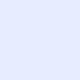# What is the formula used to measure the force that drives current?

IT Questions BankCategory: IoTWhat is the formula used to measure the force that drives current?ITExamAnswers Staff asked 4 months ago

What is the formula used to measure the force that drives current?

• I = V x R
• R = I x V
• P = I x R
• V = I x R

Explanation: Voltage is the force that drives current and is proportional to the strength of current multiplied by the resistance within a circuit.

More Questions: IoT Fundamentals Final Exam Answers Connecting Things

### Related Articles